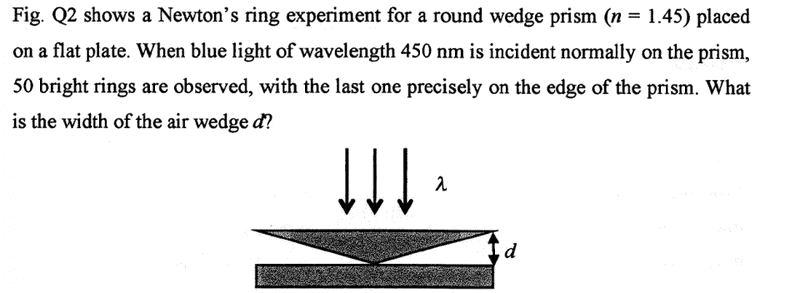kenok1216

## Homework Statementrefractive index of prism=1.45
λ=450nm
50 bright ring are observed
find d

2d=nλ/2

## The Attempt at a Solution

since 50 bright rings are observed n=24 (-24---------0-------------24)
if the distance travel=λ/2 constructive interference will happen
since the ray1 reflected by prism(n=1.45--->n=1 air)no phase shift
by ray 2 air to flat plate have phase shift

2d=24(λ/2) and λ=450nm/n=450nm/1.45=310nm
[/B]

Last edited:

drvrm

## Homework Equations

2d=nλ/2
i think
for constructive interference -draw a diagram of the rays interfering at thickness d
the correct condition perhaps is
path difference = n. wavelength (in phase)
and path difference =2.d + (wavelength / 2)

kenok1216
i think
for constructive interference -draw a diagram of the rays interfering at thickness d
the correct condition perhaps is
path difference = n. wavelength (in phase)
and path difference =2.d + (wavelength / 2)
so do you mean nλ=2d+
i think
for constructive interference -draw a diagram of the rays interfering at thickness d
the correct condition perhaps is
path difference = n. wavelength (in phase)
and path difference =2.d + (wavelength / 2)